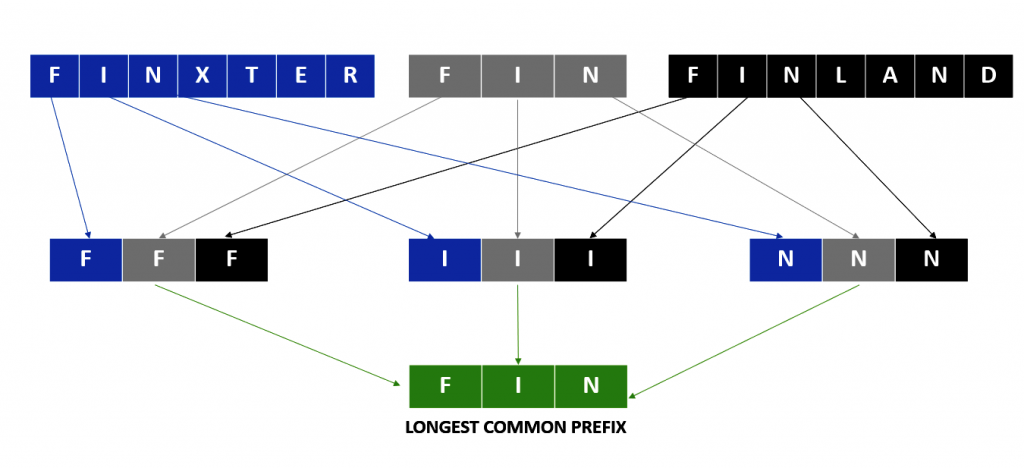# [Google Interview] How To Find The Longest Common Prefix?

Rate this post

[toc]

## Problem Statement

Write a function to find the longest common prefix string amongst an array of strings. If there is no common prefix, return an empty string “”.

Constraints:

1. `1 <= strs.length <= 200`
2. `0 <= strs[i].length <= 200`
3. `strs[i]` consists of only lower-case English letters.

## Examples

Let’s have a look at some examples to improve our understanding of this problem.

Now let’s dive into various methods to solve the problem.

## Method 1: Brute Force Approach

Approach: The naïve approach would be to check for each string and compare the characters in each string to find the common prefix.

Algorithm:

1. Initialize the first string to the “`curr`” variable.
2. If the list is empty, return an empty string.
3. If the list contains only one string, return that string.
4. For every other string in the list- Compare the character of the string with the character in the “curr” variable.
5. If the characters are equal, continue comparing, else break the loop.
6. Update the “`curr`” variable with the matched substring.

Solution:

```def common_prefix(strs):
curr = strs
if not strs:
return " "
if len(strs) == 1:
return curr
for i in range(1, len(strs)):
if len(curr) == 0:
break
temp = ''
for j in range(len(strs[i])):

if j < len(curr) and curr[j] == strs[i][j]:
temp = temp + curr[j]
else:
break

curr = temp
return curr  ```

Test Case Analysis:

Yeah! It passed all the test cases.

Complexity Analysis:

• Time Complexity: The time complexity of this method is O(n * k), where n is the size of the array and k is the size of the longest string.
• Space Complexity: O(1) as no extra space was used here.

## Method 2: Using The Two Pointer Approach

Approach: In this approach, you must sort the list of strings in lexicographical order (a to z). Then, compare the first string with the last string, character by character to find the longest common prefix.

The following illustration will help you to understand the working principle behind this approach:

In the above examplethe given array is` [Flower, Flow, Flight]`. After sorting it with the help of sort() method in Python it stores the strings in the folowing order: `[Fligth, Flow, Flower]`. Once the string has been sorted, every element/character of the first and last strings of the sorted array are compared. When a match is found it is stored in a variable (`temp`). As soon as the match is not found, the loop breaks and the value stored in the `temp` variable is returned as the output.

Algorithm:

1. Sort the given list of strings.
2. If the list is empty, return an empty string.
3. If the list contains only one string, return that string.
4. Store the first and last strings in variables “`first`” and “`last`,” respectively. Also intialize a “`temp`” variable that will store the output.
5. Compare each character of the two strings one by one.
6. If equal, add them to a temporary variable, else `break` out of the loop.
7. Finally return the temp.

Solution:

```def common_prefix(strs):
strs.sort()
if not strs:
return " "
if len(strs) == 1:
return strs

first = strs
last = strs[-1]
temp = ""
for i in range(len(first)):
if first[i] == last[i]:
temp = temp + first[i]
else:
break
return temp
```

Test case Analysis:

Yeah! It passed all the test cases.

Complexity Analysis: The time complexity of this method is O(k* n* log n), where `n` is the size of the array, `k `is the size of the longest string, and log(n) is the time taken to sort the array.

## Optimal Solution: Divide and Conquer Using zip And set

Approach: This is the most Pythonic way/approach to solve the given problem. The idea here is to divide the prefix characters of the given strings into sets of tuples and then extract the longest common prefix accordingly. Let’s have a look at the following diagram to understand this concept:Explanation: In the above example the common characters of the strings [FINXTER, FIN, FINLAD] are extracted individually within separate tuples and then each individual character is dervided from the tuple by converting it into a set and then they are merged to deduce the longest common prefix. Since a set cannot have duplicate characters, therefore as soon as the length of the set is greater than one it means you have reached the final output.

Recap to zip() Method in Python: The `zip()` function takes an arbitrary number of iterables and aggregates them to a single iterable, a zip object. It combines the i-th values of each iterable argument into a tuple. Hence, if you pass two iterables, each tuple will contain two values. If you pass three iterables, each tuple will contain three values. For example, zip together lists `[1, 2, 3]` and `[4, 5, 6]` to `[(1,4), (2,5), (3,6)]`.

Algorithm:

1. If the list is empty, return an empty string.
2. If the list contains only one string, return that string.
3. Initialize a `temp` variable that will store an empty string at the start.
4. Upon every string in the tuples generated by the `zip()` method, use the `set()` function.
5. Check the length of the set. If it is greater than 1, break the loop.
6. Else, update the `temp` to the next character.
7. Return `temp`.

Solution:

```def common_prefix(strs):
if not strs:
return " "
if len(strs) == 1:
return strs
temp = ''
for chr in zip(*strs):
if len(set(chr)) > 1:
break
else:
temp = temp + chr
return temp```

Test Case Analysis:

Yeah! It passed all the test cases.

Complexity Analysis: The time complexity of this method is O(min(k) * n), where `n` is the size of the array and `k` is the size of the shortest string.

## Conclusion

I hope you enjoyed this coding interview question. Please stay tuned and subscribe for more interesting coding problems.

Post Credits: Shubham Sayon and Rashi Agarwal